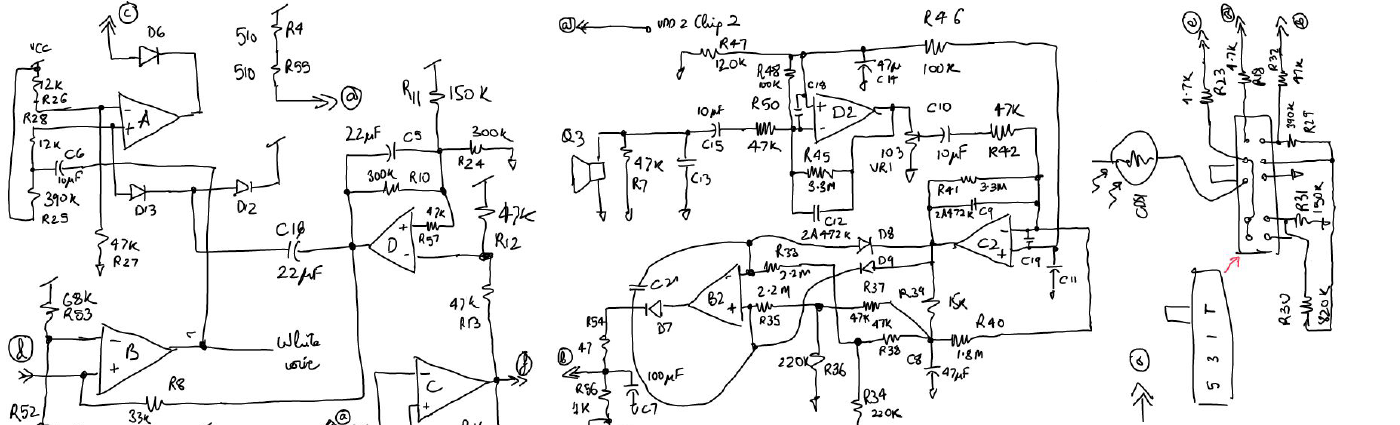# Standard Deviation difference# Problem [top]

How is the standard deviation of variation of a certain parameter (say A) of 1 physical element (say E1) related to standard deviation of the difference of parameter A between 2 different but similar physical elements (E1 and E2). In other words what is the relation between `\$σ_A\$` and `\$σ_{∆A}\$` where:

`\$σ_A\$` is the Standard Deviation of the variation of parameter A for a large sample set
`\$σ_{∆A}\$` is the Standard Deviation of the variation of the parameter `\$A_{E1}-A_{E2}\$`

# Solution [top]

Let us define some more variables:
`\$σ_{A1}\$` - Standard Deviation of the variation of parameter A for element E1
`\$σ_{A2}\$` - Standard Deviation of the variation of parameter A for element E2
`\$μ_{A1}\$` - Mean of the variation of the parameter A for element E1
`\$μ_{A2}\$` - Mean of the variation of the parameter A for element E2
`\$μ_{∆A}\$` - Mean of the variation of the parameter `\$A_{E1}-A_{E2}\$`. Note if the elements are identical then this is supposed to be 0 for the underlying process.

So we have:
`\$\$σ_{∆A}^2={∑↙{i=1}↖n[(A_{1i}-A_{2i})-μ_{∆A}]^2}/n............(1)\$\$`

where
`\$\$μ_{∆A}={∑↙{i=1}↖n(A_{1i}-A_{2i})}/n=μ_{A1}-μ_{A2}..............(2)\$\$`

Substituting this in (1) we have:
`\$\$σ_{∆A}^2={∑↙{i=1}↖n[(A_{1i}-μ_{A1})-(A_{2i}-μ_{A2})]^2}/n\$\$`

`\$\$={∑↙{i=1}↖n[(A_{1i}-μ_{A1})^2+(A_{2i}-μ_{A2})^2-2(A_{1i}-μ_{A1})(A_{2i}-μ_{A2})]}/n\$\$`

`\$\$=σ_{A1}^2+σ_{A2}^2+{∑↙{i=1}↖n[-2(A_{1i}-μ_{A1})(A_{2i}-μ_{A2})]}/n................(3)\$\$`

Now we must note a few things here:
1. For the underlying process which is being represented by these samples there is just 1 mean and standard deviation, i.e.:
`\$\$σ_{A1}=σ_{A2}=σ;μ_{A1}=μ_{A2}=μ\$\$`
So if the number of samples are large then we can assume that our mean and standard deviation represent the underlying process.
2. `\$A_{1i}\$` and `\$A_{2i}\$` are independent random variables so their mean of the product = product of their means. So now the 3rd term in equation (3) becomes:
`\$\${∑↙{i=1}↖n[-2(A_{1i}-μ_{A1})(A_{2i}-μ_{A2})]}/n=-2{∑↙{i=1}↖n(A_{1i}A_{2i}-μ(A_{1i}+A_{2i})+μ^2)}/n\$\$`
`\$\$=-2[∑↙{i=1}↖n{A_{1i}A_{2i}}/n-μ(μ+μ)+μ^2]=-2[∑↙{i=1}↖n{A_{1i}A_{2i}}/n-μ^2]\$\$`

`\$\$=-2[∑↙{i=1}↖nA_{1i}/n∑↙{i=1}↖nA_{2i}/n-μ^2]=0\$\$`

Hence equation 3 becomes:
`\$\$σ_{∆A}^2=σ_{A1}^2+σ_{A2}^2=σ^2+σ^2=2σ^2\$\$`

Thus we have:
`\$\$σ=σ_{∆A}/√2\$\$`

Or in other words the Standard Deviation of a parameter is reduced by the square root of 2 from the standard deviation of the difference of the parameter between 2 identical device samples.

This conversion is used a lot in SPICE models. SPICE generates each device's random variation independently of any other device but the mismatch is always measured by measuring the difference between 2 identical devices placed close together on the chip. So the above relation is used to translate the measured mismatch Standard Deviation to the Standard Deviation of the parameter by itself to be used in Spice models.

# Reference [top]

Below are 2 books that I use for reference. Especially the book by B. P. Lathi is really good to understand the basic concepts of communications. Highly recommend a copy for reference.
| | |